Courses
Courses for Kids
Free study material
Free LIVE classes
MoreLIVE
Join Vedantu’s FREE Mastercalss

What is the first thing that crosses your mind when you think of Maths? Is it a number? Is it a date? Or is it some long calculation? Well, even though the word ‘Maths’ brings in a cloud of calculations and random numbers, there is definitely something more interesting and less tedious than the long calculations to it. There is a long list of special Maths words knowing which you can ace the concepts and theorems of Maths easily. The below-given Maths words that start with S will equip you with an upper hand at Maths.

## List of Maths Words That Begin with S

• Sum: When we add two or more quantities, the result obtained is called the sum. For example, 2+5=7. Here, 7 is the sum.

• Subtract: It is a Mathematical operation that involves taking away a definite quantity or number from a given quantity or number. For example, subtracting 10 from 25, we obtain 25-10 =15.

• Scale: It is a conventional ratio of the length or distance in a model or map to the real length or distance. For example, if we depict a distance of 10 kilometres on the ground as a distance of 1 kilometre on a map, then we take a scale of 1:10.

• Square: It is a 2D plane figure, enclosed by 4 straight sides. All the sides of this shape are equal in length and each interior angle measures 900. As it has 4 sides, a square is called a quadrilateral.

• Segment: A straight line that connects two points in space is called a segment.

• Symmetry: When an object can be split into two equal halves, about an imaginary axis, such that each half is a mirror image of the other, this correspondence is called symmetry.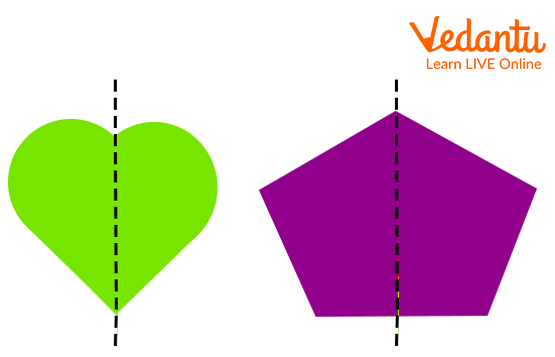Image of examples of symmetry

• Symbol: A pattern, sign, character, or image that is used to represent a word or operation in Maths is called a symbol. For example, ‘%’ is the symbol for percentage.

• Semi-Circle: When a circle is divided into two equal parts by its diameter, each part is called a semi-circle. The radius of a semicircle is the same as that of the circle. The circumference, area, and perimeter of the semi-circle are obtained by dividing the circumference, area, and perimeter of the circle by 2.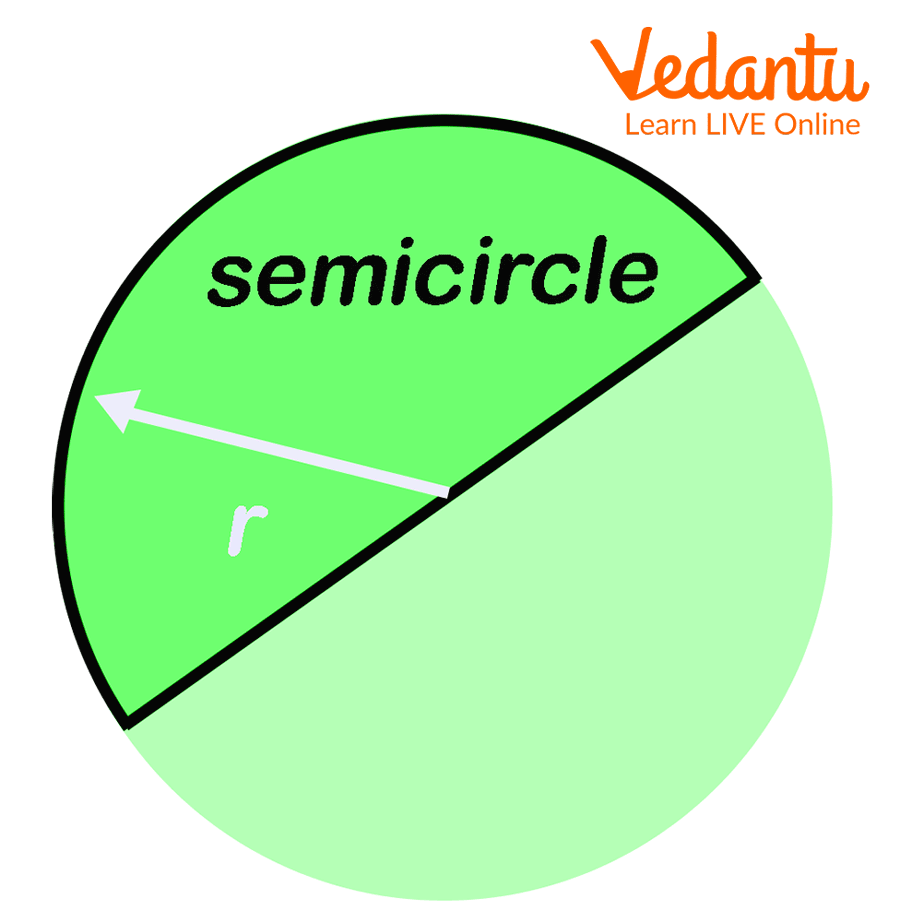Image of a semicircle

• Sphere: It is a 3D geometric figure that resembles a ball. Every point on a sphere is at the same distance from its centre.

• Speed: Speed is the measure of how fast an object moves. It is calculated by dividing the distance covered by an object by the time taken to cover the distance. Therefore, speed is defined as the distance covered by an object in unit time. The units used to measure this quantity are metre/second and kilometre/hour.

• Surface Area: The total area covered by all the surfaces of a 3D object is called its surface area. For example, the surface area of a cuboid is calculated by adding up the surface area of each of its surfaces.

• Sector: A sector is the part of a circle formed when two of its radii connect at the centre. It looks like a pie slice or slice of a pizza.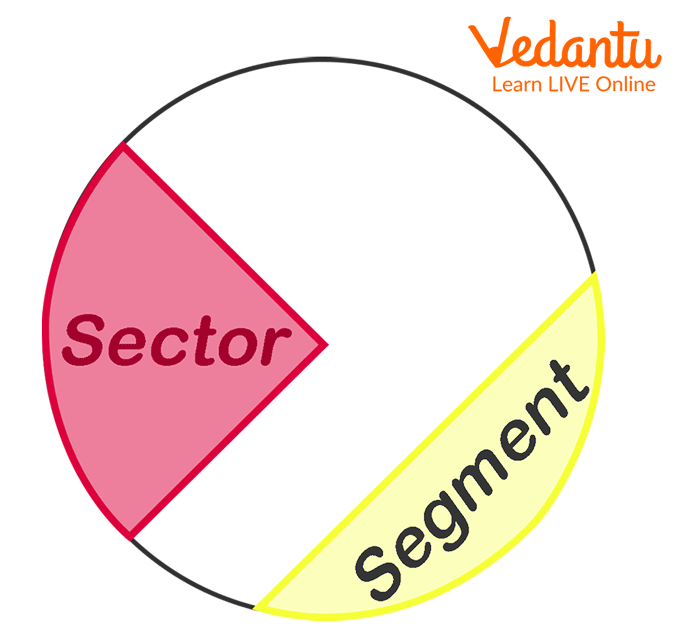Image of a circle showing its sector and segment

• Square Root: The square root of a given number refers to the value which when multiplied by itself yields the original number. For example, 25= 5 x 5.

• Sine: The sine of an angle in a right-angled triangle is given as the ratio of the length of the side opposite to the angle to the hypotenuse of the triangle. It is abbreviated as ‘sin’.

Sin θ = perpendicular ÷ hypotenuse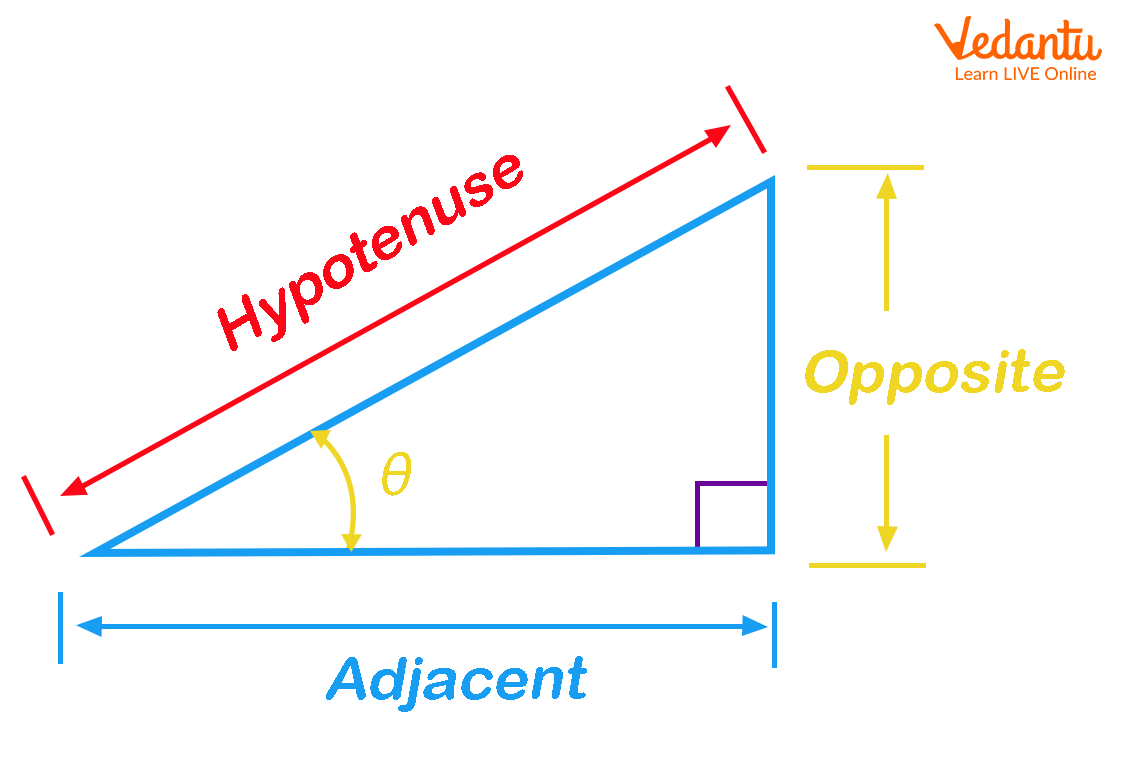Image of a right-angled triangle

• Simple Interest: It is the interest calculated on the principal amount by multiplying the principal amount, percent or rate of interest, and time. It is abbreviated as S.I. and expressed as S.I.= P x R x T/100

• Standard Deviation: It is a measure of how distributed or spread out the values of a data set are. It is calculated by taking a square root of Variance.

(Variance is calculated as the average of squared differences of the values from the Mean.)

• Surd: When a number contains square roots or cube roots that cannot be adjusted and removed to simplify the number, it is called a surd. For example, the $\sqrt{3}$ is a surd whereas $\sqrt{9}$ is not a surd.

• Slant Length: For a cone, the length of its slanting sides is called the slant length. It is abbreviated as ‘s’. Refer to the image given below to find that the slant length of a cone can be calculated as s = $\sqrt{(h^{2}+r^{2})}$. Slant length is also observed in pyramids.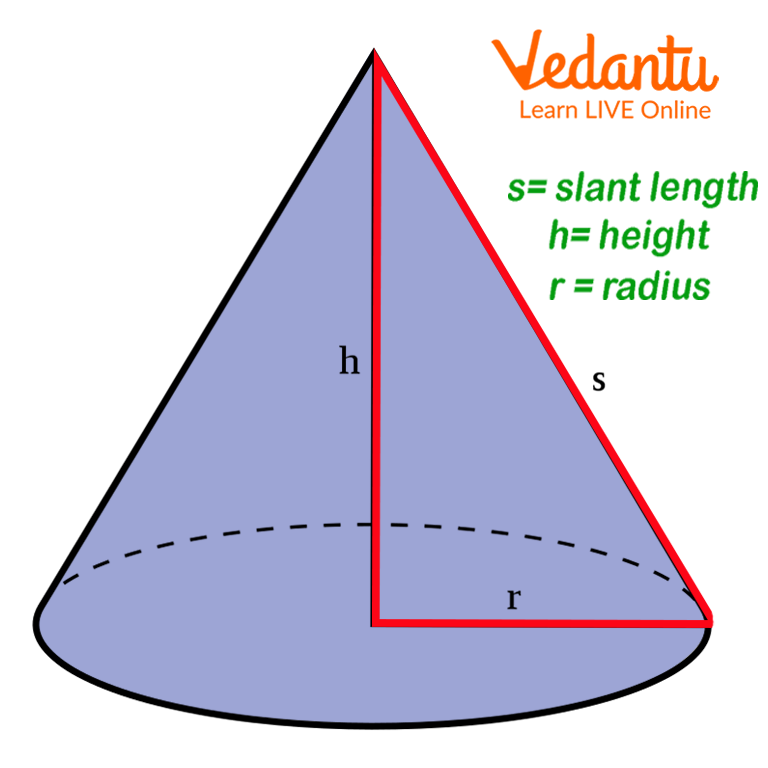Image of a cone

• Set: A finite or infinite collection of unique numbers, quantities, objects, or things is called a set. Each member of a set is called its element and the elements of a set are enclosed within the curly brackets. For example, {2, 4, 6, 8, 10} is a set of even numbers from 1 to 10.

• Sample Space: It is the set of all outcomes possible when an event occurs. For example, if a coin is flipped in the air, the sample space will have only two possible outcomes. The sample space with all the possible outcomes of this event will be:

S={H, T}.

• Superset: When a set comprises all the elements of other smaller sets, it is referred to as the superset of the smaller sets. For example:

Set A = {1, 2, 3, 4, 5, 6, 7, 8, 9, 10, 11, 12, 13, 14, 15, 16, 17, 18, 19, 20}

Set B = {2, 4, 6, 8, 10, 12, 14, 16, 18, 20}

Set C = {5, 10, 15, 20}

Here, Set A is the superset of Set B and Set C.

Every set is a super set of the empty set.

• Subset: If there are two sets Set A and Set B such that Set A comprises all the elements of Set B, then Set B is referred to as the subset of Set A.

Example:

Set A ={ 10, 20, 30, 40, 50, 60, 70, 80, 90, 100}

Set B = {20, 40, 60, 80, 100}

Here, Set B is the subset of Set A.

• Supplementary Angles: When the sum of two angles measures up to 180 degrees, the angles are called supplementary angles. A pair of supplementary angles may or may not be on a straight line. For example, 110° + 70 ° = 180°, therefore, 110 ° and 70° are supplementary angles

• Sales Tax: Sales tax is an additional sum of money charged on the selling price of goods/commodities, or services. In general, it is paid to the national government of our country. For example, if you buy a tshirt for 400 INR, and the sales tax charged on it 5%, you pay 20 INR as the sales tax.

## Conclusion

There are many more Maths words that start with S apart from the above list of words. However, the list of words discussed above are some of the most used Maths words and students will surely come across them in their Maths chapters. To ease out the learning process for them, these simple Maths words that start with S can be taught with the help of pictorial representations. Also check out the common Maths words that start with other alphabets like V, G, E, P, etc. on Vedantu and help your kids with approaching the theories of Mathematics in a unique yet effective way.

Last updated date: 29th Sep 2023
Total views: 135.6k
Views today: 1.35k

1. What are the five most commonly used Maths words that start with S?

The five most commonly used Maths words that begin with the letter S are as follows:

• Sum

• Subtract

• Square

• Scalene Triangle

• Set

2. How is speed calculated?

Speed is calculated by dividing the distance travelled by car, bus, train, or any other moving object, by the time the object took to cover the distance. It is expressed in units of distance/time, that is, metres/second or kilometres/hour.